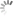## 资源 | 概率编程工具：TensorFlow Probability官方简介

x

选自Medium

作者：Josh Dillon、Mike Shwe、Dustin Tran

机器之心编译

参与：白妤昕、李泽南

TensorFlow Probability 适用于以下需求：

希望建立一个生成数据模型，推理其隐藏进程。

需要量化预测中的不确定性，而不是预测单个值。

训练集具有大量相对于数据点数量的特征。

TensorFlow Probability 可以解决这些问题。它继承了 TensorFlow 的优势，例如自动差异化，以及跨多种平台（CPU，GPU 和 TPU）性能拓展能力。

TensorFlow Probability 有哪些能力？

谷歌的机器学习概率工具为 TensorFlow 生态系统中的概率推理和统计分析提供模块抽象。TensorFlow Probability 的结构示意图。概率编程工具箱为数据科学家和统计人员以及所有 TensorFlow 用户提供便利。

第 0 层：TensorFlow。数值运算。LinearOperator 借助特殊结构（对角线，低秩等）进行高效计算，而不再借助矩阵。它由 TensorFlow Probability 团队构建和维护，现在已经是 TensorFlow 核心 tf.linalg 的一部分

第 1 层：统计构建模块

分布（tf.contrib.distributions，tf.distributions）：包含大量概率分布和相关的统计数据，以及批量语义和广播语义。

第 2 层：模型构建

Edward2（tfp.edward2）：这是一种指定灵活的概率模型为程序的概率编程语言。

概率层（tfp.layers）：它们所代表的功能对神经网络层具有不确定性，扩展了 TensorFlow 图层。

可训练分布（tfp.trainable_distributions）：由单个张量参数化的概率分布，我们更容易建立输出概率分布的神经网络。

第 3 层：概率推断

马尔可夫链蒙特卡罗方法（tfp.mcmc）：通过采样近似积分的算法。包括 Hamiltonian Monte Carlo（HMC 算法），随机过程 Metropolis-Hastings，以及构建自定义过渡内核的能力。

变分推理（tfp.vi）：通过优化来近似积分的算法。

优化器（tfp.optimizer）：随机优化方法，扩展 TensorFlow 优化器。包括随机梯度 Langevin 动态。

蒙特卡罗（tfp.monte_carlo）：用于计算蒙特卡罗期望值的工具。

第 4 层：预制模型和推理（类似于 TensorFlow 的预制估算器）

贝叶斯结构时间序列（即将推出）：用于拟合时间序列模型的高级接口（即类似于 R 的 BSTS 包）。

广义线性混合模型（即将推出）：用于拟合混合效应回归模型的高级界面（即与 R 的 lme4 软件包相似）。

TensorFlow Probability 团队致力于通过最新的功能，持续代码更新和错误修复来支持用户和贡献者。谷歌称，该工具在未来会继续添加端到端的示例和教程。

让我们看看一些例子！

Edward2 的线性混合效应模型

线性混合效应模型是对数据中结构化关系进行建模的简单方法。也称为分级线性模型，它分享各组数据点之间的统计强度，以便改进对任何单个数据点的推论。

演示中考虑到 R 语言中流行的 lme4 包里的 InstEval 数据集，其中包含大学课程及其评估评级。使用 TensorFlow Probability，我们将模型指定为 Edward2 概率程序（tfp.edward2），该程序扩展了 Edward。下面的程序根据其生成过程来确定模型。

`import tensorflow as tffrom tensorflow_probability import edward2 as eddef model(features): # Set up fixed effects and other parameters. intercept = tf.get_variable("intercept", []) service_effects = tf.get_variable("service_effects", []) student_stddev_unconstrained = tf.get_variable( "student_stddev_pre", []) instructor_stddev_unconstrained = tf.get_variable( "instructor_stddev_pre", []) # Set up random effects. *student_effects = ed.MultivariateNormalDiag( loc=tf.zeros(num_students), scale_identity_multiplier=tf.exp( student_stddev_unconstrained), name="student_effects") instructor_effects = ed.MultivariateNormalDiag( loc=tf.zeros(num_instructors), scale_identity_multiplier=tf.exp( instructor_stddev_unconstrained), name="instructor_effects")* # Set up likelihood given fixed and random effects. *ratings = ed.Normal( loc=(service_effects * features["service"] + tf.gather(student_effects, features["students"]) + tf.gather(instructor_effects, features["instructors"]) + intercept), scale=1., name="ratings")*return ratings`

该模型将「服务」、「学生」和「教师」的特征字典作为输入，它对每个元素描述单个课程的向量。模型会回归这些输入，假设潜在的随机变量，并返回课程评估评分的分布。在此输出上运行的 TensorFlow 会话将返回 yigediedai 一个迭代的评分。

你可以查看「线性混合效应模型」教程，详细了解如何使用 tfp.mcmc.HamiltonianMonteCarlo 算法训练模型，以及如何使用后验预测来探索和解释模型。

高斯 Copulas 与 TFP Bijectors

Copula 是多变量概率分布，其中每个变量的边际概率分布是均匀的。要构建使用 TFP 内在函数的 copula，可以使用 Bijectors 和 TransformedDistribution。这些抽象可以轻松创建复杂的分布，例如：

`import tensorflow_probability as tfptfd = tfp.distributionstfb = tfp.distributions.bijectors# Example: Log-Normal Distributionlog_normal = tfd.TransformedDistribution( distribution=tfd.Normal(loc=0., scale=1.), bijector=*tfb.Exp*())# Example: Kumaraswamy DistributionKumaraswamy = tfd.TransformedDistribution( distribution=tfd.Uniform(low=0., high=1.), bijector=*tfb.Kumaraswamy*( concentration1=2., concentration0=2.))# Example: Masked Autoregressive Flow# https://arxiv.org/abs/1705.07057shift_and_log_scale_fn = *tfb.masked_autoregressive_default_template*( hidden_layers=[512, 512], event_shape=[28*28])maf = tfd.TransformedDistribution( distribution=tfd.Normal(loc=0., scale=1.), bijector=*tfb.MaskedAutoregressiveFlow*( shift_and_log_scale_fn=shift_and_log_scale_fn))`

「高斯 Copula」创建了一些自定义的 Bijectors，然后展示了如何轻松构建多个 copula。有关分布的更多背景信息，请参阅「了解张量流量分布形状」一节。其中介绍了如何管理抽样，批量训练和建模事件的形状。

带有 TFP 实用工具的变分自编码器

变分自编码器是一种机器学习模型，使用一个学习系统来表示一些低维空间中的数据，并且使用第二学习系统来将低维数据还原为原本的输入值。由于 TensorFlow 支持自动微分，因此黑盒变分推理是一件轻而易举的事！

示例：

`import tensorflow as tfimport tensorflow_probability as tfp# Assumes user supplies `likelihood`, `prior`, `surrogate_posterior`# functions and that each returns a # tf.distribution.Distribution-like object.elbo_loss = *tfp.vi.monte_carlo_csiszar_f_divergence( *f=*tfp.vi.kl_reverse*, # Equivalent to "Evidence Lower BOund" p_log_prob=lambda z: likelihood(z).log_prob(x) + prior().log_prob(z), q=surrogate_posterior(x), num_draws=1)train = tf.train.AdamOptimizer( learning_rate=0.01).minimize(elbo_loss)`

要查看更多详细信息，请查看我们的变分自编码器示例！

具有 TFP 概率层的贝叶斯神经网络

贝叶斯神经网络是一个在其权重和偏倚上具有先验分布的神经网络。它通过这些先验提供了更加先进的不确定性。贝叶斯神经网络也可以解释为神经网络的无限集合：分配给每个神经网络配置的概率是有先验根据的。

作为演示，考虑具有特征（形状为 32 × 32 × 3 的图像）和标签（值为 0 到 9）的 CIFAR-10 数据集。为了拟合神经网络，我们将使用变分推理，这是一套方法来逼近神经网络在权重和偏差上的后验分布。也就是说，我们使用 TensorFlow Probabilistic Layers 模块（tfp.layers）中最近发布的 Flipout 估计器。

`import tensorflow as tfimport tensorflow_probability as tfpdef neural_net(inputs): net = tf.reshape(inputs, [-1, 32, 32, 3]) *net = tfp.layers.Convolution2DFlipout(filters=64, kernel_size=5, padding='SAME', activation=tf.nn.relu)(net)* net = tf.keras.layers.MaxPooling2D(pool_size=2, strides=2, padding='SAME')(net) net = tf.reshape(net, [-1, 8 * 8 * 64]) *net = tfp.layers.DenseFlipout(units=10)(net)* return net# Build loss function for training.logits = neural_net(features)neg_log_likelihood = tf.nn.softmax_cross_entropy_with_logits( labels=labels, logits=logits)kl = sum(tf.get_collection(tf.GraphKeys.REGULARIZATION_LOSSES))loss = neg_log_likelihood + kltrain_op = tf.train.AdamOptimizer().minimize(loss)`

neural_net 函数在输入张量上组成神经网络层，并且针对概率卷积层和概率密集连接层执行随机前向通道。该函数返回具有批大小 10 的形状的输出张量。张量的每一行代表每个数据点属于 10 个类别之一的 logits（无约束概率值）。

我们需要为训练建立损失函数，它包括两个项：预期的负对数似然和 KL 分歧。我们可以通过蒙特卡罗接近预期的负的 log 似然函数。KL 分歧是通过作为层的参数的正规化术语添加的。

tfp.layers 也可以用于使用 tf.keras.Model 类的 eager execution。

`class MNISTModel(tf.keras.Model): def __init__(self): super(MNISTModel, self).__init__() *self.dense1 = tfp.layers.DenseFlipout(units=10) self.dense2 = tfp.layers.DenseFlipout(units=10)* def call(self, input): """Run the model.""" result = self.dense1(input) result = self.dense2(result) # reuse variables from dense2 layer result = self.dense2(result) return resultmodel = MNISTModel()`

快速上手

请运行如下链接，开始使用 TensorFlow 中的概率机器学习：

`pip install --user --upgrade tfp-nightly`

对于所有的代码和细节，请查看 github.com/tensorflow/probability。谷歌希望能够通过 GitHub 与所有开发者展开合作。

原文链接：https://medium.com/tensorflow/introducing-tensorflow-probability-dca4c304e245

本文为机器之心编译，转载请联系本公众号获得授权

------------------------------------------------

加入机器之心（全职记者/实习生）：hr@jiqizhixin.com

投稿或寻求报道：editor@jiqizhixin.com

广告&商务合作：bd@jiqizhixin.com

Notice: The content above (including the pictures and videos if any) is uploaded and posted by a user of NetEase Hao, which is a social media platform and only provides information storage services.

© 1997-2020 网易公司版权所有 About NetEase | 公司简介 | 联系方法 | 招聘信息 | 客户服务 | 隐私政策 | 广告服务 | 网站地图 | 意见反馈 | 不良信息举报

#### 机器之心Pro#### 机器之心Pro

4322

94927列表加载中...

x